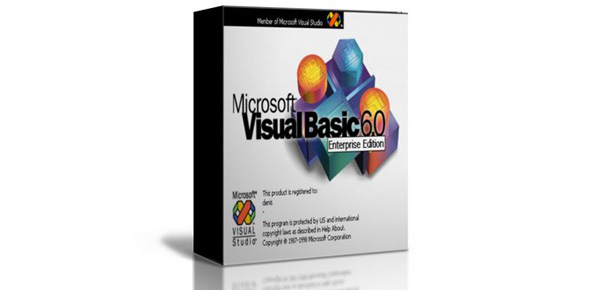# Visual Basic - Chapter 4 Quiz

20 QuestionsSettingsVisual Basic. NET Chapter 4 Quiz - Programming 11

Related Topics
• 1.
A variable is a named memory location that stores a value.
• A.

True

• B.

False

• 2.
A numeric variable is automatically initialized to 1 in Visual Basic .NET.
• A.

True

• B.

False

• 3.
A Single is a numeric value that can contain a decimal portion.
• A.

True

• B.

False

• 4.
Multiple variables with the same data type can be declared in a single statement.
• A.

True

• B.

False

• 5.
A variable can store more than one value at any given time.
• A.

True

• B.

False

• 6.
An example of a Visual Basic .NET keyword is Single.
• A.

True

• B.

False

• 7.
Boolean variables are initialized to True.
• A.

True

• B.

False

• 8.
String variables are initialized to Nothing.
• A.

True

• B.

False

• 9.
A value with a decimal portion is automatically rounded to a whole number when assigned to an Integer variable.
• A.

True

• B.

False

• 10.
A local declaration allows a variable to be used anywhere in the module or form.
• A.

True

• B.

False

• 11.
Which object allows users to enter values?
• A.

A Label object

• B.

A Button object

• C.

A TextBox object

• D.

• 12.
A label placed near a text box that describes its contents or purpose is called a
• A.

Text box label.

• B.

Prompt.

• C.

Question.

• D.

Content label.

• 13.
The TextBox control Name property
• A.

Sets the alignment of the text relative to the text box.

• B.

Sets the alignment of the text relative to the form.

• C.

Determines what text is displayed in the text box.

• D.

Identifies a control for the programmer.

• 14.
The TextBox control Text property
• A.

Sets the alignment of the text relative to the text box.

• B.

Sets the alignment of the text relative to the form.

• C.

Determines what text is displayed in the text box.

• D.

Identifies a control for the programmer.

• 15.
A procedure that performs a task and returns a value is called
• A.

An assignment

• B.

a text changed event.

• C.

A function.

• D.

An event procedure.

• 16.
A named memory location which stores a value that cannot be changed from its initial assignment is called a
• A.

Constant.

• B.

Variable.

• C.

Procedure.

• D.

Function.

• 17.
Which data type should be used to represent values with a decimal portion?
• A.

Single

• B.

Integer

• C.

Short

• D.

Boolean

• 18.
Which data type should be used to represent single characters?
• A.

Boolean

• B.

Char

• C.

String

• D.

Long

• 19.
Misspelling the word Integer would result in which type of error?
• A.

Syntax error

• B.

Logic error

• C.

Dynamic error

• D.

Run-time error

• 20.
Which type of error produces undesired or unexpected results?
• A.

Syntax error

• B.

Logic error

• C.

Dynamic error

• D.

Run-time error